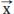NextPrevious

# What is the component of a vector?

A component of a vector is one with n numbers in a certain order. It is usually listed as (x1, x2, …, xn), in which the numbers within the parentheses are called the components of the vector.

Logically, an infinite number of vectors can have the same components. For example, if the components are [3, 4], we know there are an infinite number of pairs of points in the plane with x and y coordinates whose respective differences are 3 and 4. All these vectors are parallel to each other, equal, and have the same magnitude and direction. Therefore, any vector with components of a and b can be said to be equal to the vector [a, b].

Close

This is a web preview of the "The Handy Math Answer Book" app. Many features only work on your mobile device. If you like what you see, we hope you will consider buying. Get the App